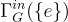### Structure of intersection graphs

Haval M. Mohammed Salih, Sanaa M. S. Omer

#### Abstract

Let G be a finite group and let N be a fixed normal subgroup of G.  In this paper, a new kind of graph on G, namely the intersection graph is defined and studied. We useto denote this graph, with its vertices are all normal subgroups of G and two distinct vertices are adjacent if their intersection in N. We show some properties of this graph. For instance, the intersection graph is a simple connected with diameter at most two. Furthermore we give the graph structure offor some finite groups such as the symmetric, dihedral, special linear group, quaternion and cyclic groups.

#### Keywords

connected graph, normal subgroup, diameter

#### Full Text:

PDF

DOI: http://dx.doi.org/10.19184/ijc.2021.5.2.6

#### References

Tong-Viet, Hung P, Finite groups whose prime graphs are regular, J. Algebra, 397 (2014), 18-31.

Kurzweil, Hans and Stellmacher, Bernd, The theory of finite groups: an introduction, Springer Science & Business Media, (2006).

Wilson, Robin J, Introduction to graph theory, Pearson Education India, (1979).

Erfanian, Ahmad and Tolue, Behnaz, Conjugate graphs of finite groups, Discrete Math. Algorithms Appl., 4(02), (2012).

Hai, Zhang Qin and Ji, Cao Jian, Finite groups whose nontrivial normal subgroups have the same order, J. Math. Res. Exp., 28(4), (2008), 807-812.

### Refbacks

• There are currently no refbacks.

ISSN: 2541-2205

View IJC Stats